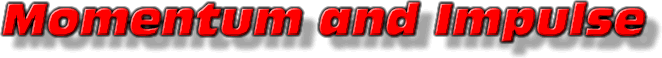This text is meant to accompany class discussions. It is not everything there is to know about energy. It is meant as a  prep for class. More detailed notes and examples are given in the class notes, presentations, and demonstrations (click here.)
Impulse

The process of transferring momentum is called impulse.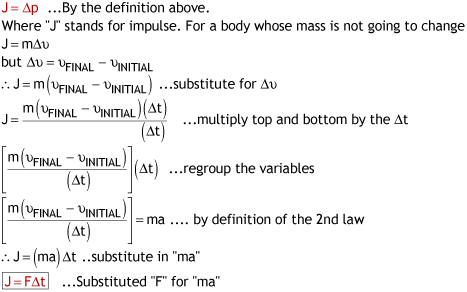When a force is applied for period of time an impulse is delivered. Looking at the derivation you can combine two of the expressions for impulse to get a familiar relationship.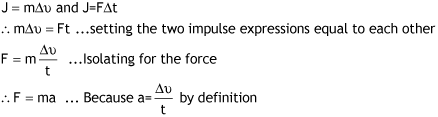Impulse comes from Newton's 3rd law of motion.

When solving word problems, remember that the impulse to start an object moving from rest is equal and opposite to the impulse needed to stop that same object. This is a helpful problem solving strategy.

 A car with a mass of exactly 1000 kg starts from rest and travels to 25 m/s in 10.0 seconds. After traveling some distance at a constant speed the car applies the brakes and slows down to a stop in 5.0 seconds. Use impulse to find out how much force was used to stop the car. Click here to see an image of the solution in case you cannot get access to YouTube.

This equation works because the force is constant. If the force changes over time, a graph will be used to determine the impulse instead of the product of "Ft."

If the force that changes the momentum of an object is applied over time then a graph can be plotted to calculate the impulse.

This method provides one more way to calculate the impulse. Impulse can be calculated by the formulas, J=m(change-in-v) and J=Ft and the areas between the curve and axis.

Example

 Example A 1400 kg car accelerate in in first gear following the force vs time graph shown below. If the car starts from rest, What is its final speed after 2 seconds?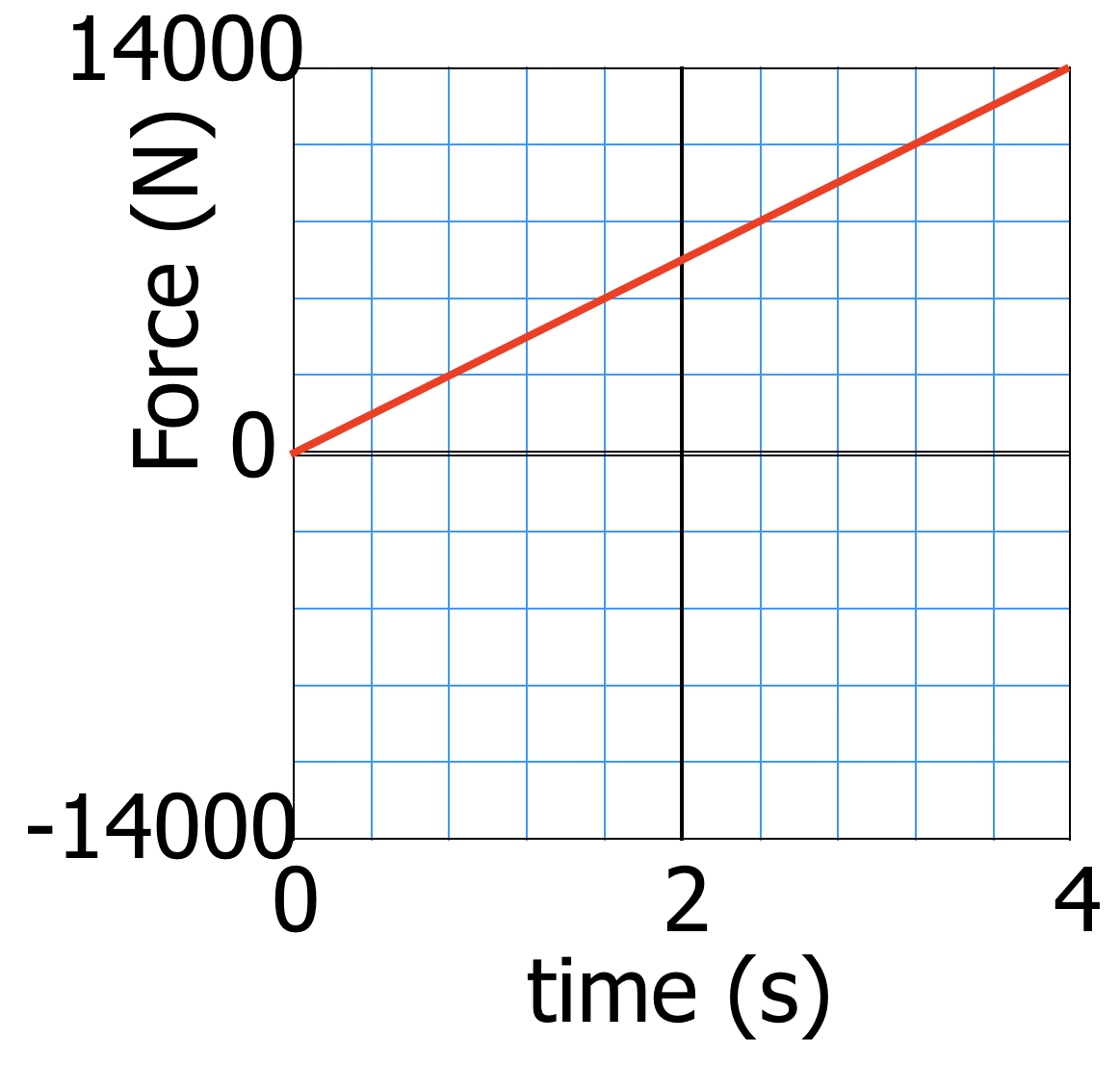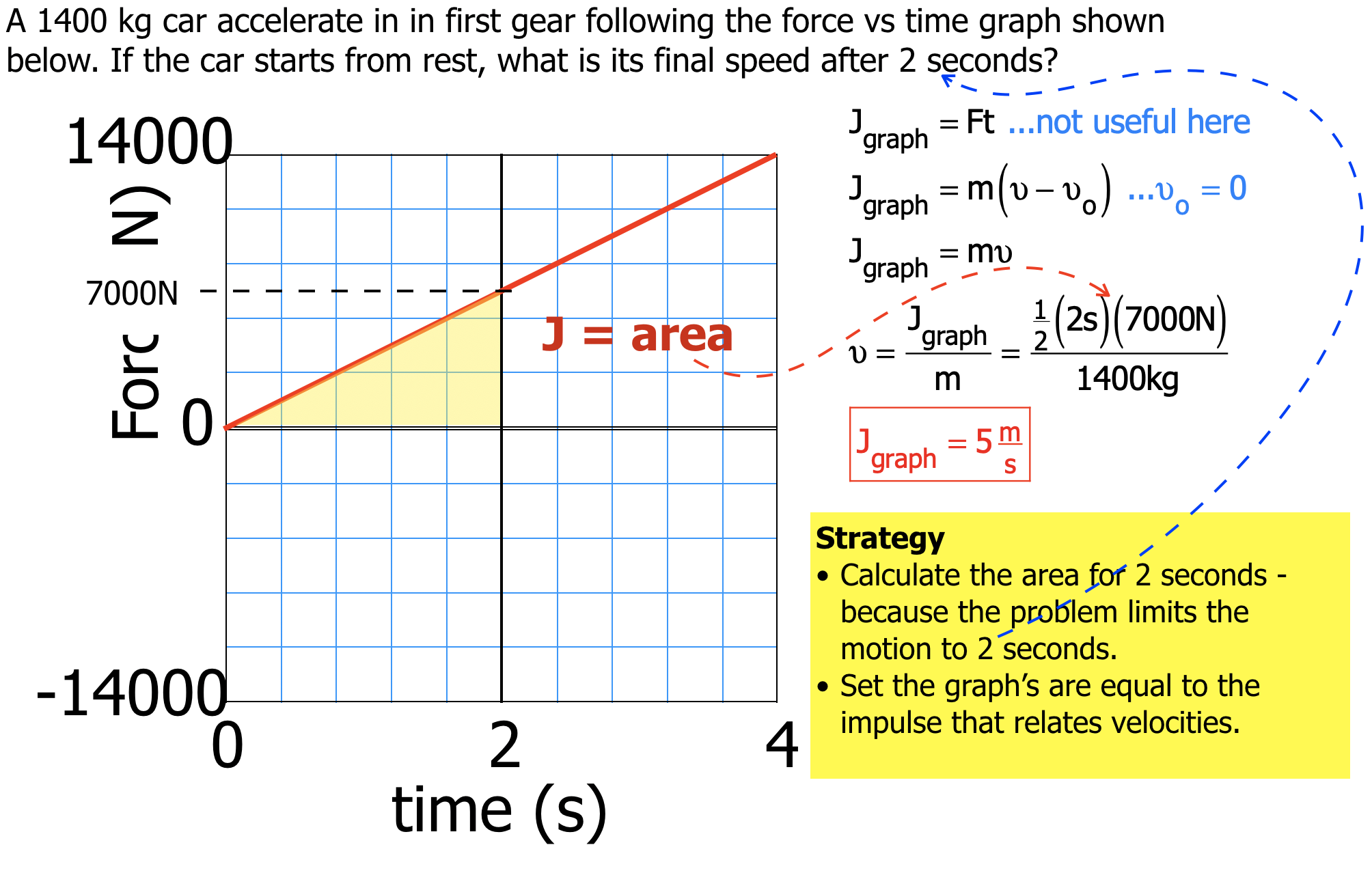Conservation of Momentum

The law of conservation of momentum says In the absence of outside force, the momentum of a system must not change.

A "collision" occurs whenever momentum is exchanged between two or more bodies who are approaching each other. For collisions the law of conservation of momentum can expressed one of two ways.

1. The sum of each body's momentum before a collision equals the sum of each body's momentum after a collision.
2. During a collision/interaction, the momentum lost by one body equals the momentum gained by another.

Mathematically these two laws look like this:This section will look mathematically at the first expression for solving problems. It is equally correct to use the second expression.

Perfectly Elastic Collisions and the Conservation of Momentum

Momentum is a vector. So vector addition can be used to see the 1st law of conservation of momentum. Try the example below to see how vectors can be used. Remember, adding up the vectors associated with the bodies' momentums before the collision equals the adding up the momentum vectors after the collision.

by Tony Wayne ...(If you are a teacher, please feel free to use these resources in your teaching.)

 The owner of this website does not collect cookies when the site is visited. However, this site uses and or embeds Adobe, Apple, GoDaddy, Google, and YouTube products. These companies collect cookies when their producs are used on my pages. Click here to go to them to find out more about how they use their cookies. If you do not agree with any of their policies then leave this site now.